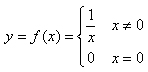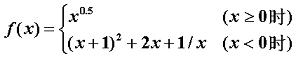# 7-1 简单题 (5分)

#include<stdio.h>
int main()
{
printf("This is a simple problem.");
}


# 7-2 将x的平方赋值给y (5分)

y = x * x

x * x = y

#include<stdio.h>

int main(int argc, char *argv[]) {
printf("9 = 3 * 3\n3 * 3 = 9");
return 0;
}


# 7-3 A乘以B (10分)

-8 13

-104

#include <stdio.h>
int main()
{
int A,B;
scanf("%d %d",&A,&B);
printf("%d\n",A*B);
return 0;
}


# 7-4 是不是太胖了 (5分)

169

124.2

#include<stdio.h>
int main ()
{
double H,weight ;
scanf("%lf",&H);
weight=(H-100)*0.9*2;
printf("%.1f\n",weight);
return 0;
}


# 7-5 输出各位数字 (10分)

258

2,5,8

#include<stdio.h>
int main()
{
int n;
scanf("%d",&n);
printf("%d,%d,%d",n/100,(n/10)%10,n%10);
return 0;
}


# 7-6 整数四则运算 (10分)

3 2

3 + 2 = 5

3 - 2 = 1

3 * 2 = 6

3 / 2 = 1

#include<stdio.h>
int main()
{
int A, B;
scanf("%d %d", &A, &B);
printf("%d + %d = %d\n", A, B, A + B);
printf("%d - %d = %d\n", A, B, A - B);
printf("%d * %d = %d\n", A, B, A * B);
printf("%d / %d = %d\n", A, B, A / B);
}


# 7-7 计算银行存款的本息 (5分)

1000 3 0.025

sum = 1076.89

#include <stdio.h>
#include "math.h"

int main(int argc, char *argv[]) {
int money,year;
float rate;
float sum = 0;
scanf("%d %d %f",&money,&year,&rate);
sum = money*pow((1+rate),year);
printf("sum = %.2f",sum);
}


pow 函数

# 7-9 计算分段函数 (10分)10

f(10.0) = 0.1

0

f(0.0) = 0.0

#include <stdio.h>
int main(int argc, char *argv[]) {
float x=0.0;
scanf("%f",&x);
if(x!=0)
printf("f(%.1f) = %.1f",x,1/x);
if(x==0)
printf("f(0.0) = 0.0");
}


# 7-10 计算分段函数 (10分)10

f(10.00) = 3.16

-0.5

f(-0.50) = -2.75

#include <stdio.h>
#include "math.h"
int main(int argc, char *argv[]) {

float x=0.0;
scanf("%f",&x);
if(x>=0)
printf("f(%.2f) = %.2f",x,pow(x,0.5));
if(x<0)
printf("f(%.2f) = %.2f",x,pow(x+1,2)+2*x+1/x);
}


# 7-11 偶数乘2奇数乘3 (10分)

3

y=9

2

y=4

#include <stdio.h>
int main(int argc, char *argv[]) {
int x=0;
scanf("%d",&x);
if(x%2==0)
printf("y=%d",x*2);
if(x%2!=0)
printf("y=%d",x*3);
}


# 7-12 整除问题 (10分)

21 7

YES

#include <stdio.h>

int main(int argc, char *argv[]) {
int x=0;
int y=0;
scanf("%d %d",&x,&y);
if(x%y==0)
printf("YES");
else
printf("NO");

}